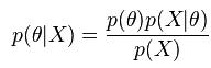Explore BrainMass

# Posterior Distribution

Contrary to the prior, the posterior distribution represents the distribution for the unknown quantity or parameter of interest, after the observed or necessary data has been applied. When calculating the posterior probability, Bayes’ Theorem can be utilized for calculating the distribution for one unknown, random variable.

After collecting the observed data, the posterior distribution is a representation of the distribution of parameters, including the uncertain parameters, which is based from the most current evidence. Essentially, the posterior distribution represents a method for summarizing what is known for current uncertain quantities.

Additionally, the posterior distribution can be summarized in multiple ways. This includes being expressed as the posterior median, the posterior expectation and the posterior probability.

The posterior probability can be defined using the following expression:Variables:

p(θ) = probability distribution function

X = observations

p(X|θ) = likelihood

In a basic sense, the posterior distribution can be thought of as a medium between the prior distribution and the observed data. Overall, the posterior distribution represents the basis for statistical inference. Furthermore, eventually the posterior distribution will become the prior distribution if a newer investigation or model is needed requiring further data.